﻿ 基于矩阵循环的智能RGV的动态调度策略 Dynamic Scheduling Strategy of Smart RGV Based on Matrix Cycle

Vol. 08  No. 03 ( 2019 ), Article ID: 29287 , 15 pages
10.12677/AAM.2019.83054

Dynamic Scheduling Strategy of Smart RGV Based on Matrix Cycle

Yang Gu*#, Zhourong Zhang, Jin Jiang

College of Science, Nanjing University of Aeronautics and Astronautics, Nanjing Jiangsu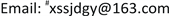Received: Feb. 28th, 2019; accepted: Mar. 12th, 2019; published: Mar. 19th, 2019ABSTRACT

This paper mainly studies the dynamic scheduling problem of a smart RGV. For the processing operation of one process, it is judged by simulation analysis that the cyclic cycle scheduling is the optimal scheduling. Therefore, we establish an optimal scheduling model based on matrix cycle, and transform the path planning into a traveling salesman problem (TSP). Then we use Lingo and MATLAB to solve three sets of parameters, the total number of workpiece production is 384, 347, and 395, respectively, and the system operation efficiency is as high as 98%. For the two processes, the time determinant y is introduced to represent the ratio of the time required for the two processes. We analyze in three cases: y is approximately 1, y is greater than 1 and y is less than 1, and respectively establish optimal scheduling model based on time determinant. The total number of workpieces produced under the three parameters of the solution is 243, 226, 295, and the system operating efficiency is 88%, 82%, and 89%, respectively. For the fault situation, the time is divided into several segments according to the fault point, and we establish the optimal scheduling model for fault reduction dimension based on time segmentation. The total number of workpieces produced in one process is 370, 322, and 378, and the system operation efficiency is as high as 94%, 91%, and 94%. The total number of workpieces produced in two processes is 212, 198, and 250, respectively, and the system operation efficiency is 77%, 72%, and 75%, respectively.

Keywords:Matrix Cycle, Traveling Salesman Problem, Time Determinant, Fault Reduction Dimension, Optimal Scheduling1. 引言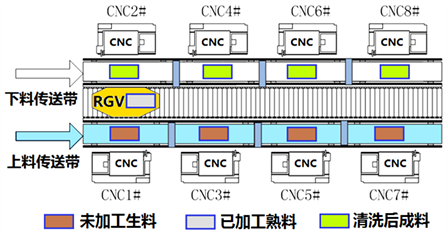Figure 1. Schematic diagram of smart processing system

1) 一道工序的物料加工作业情况，每台CNC安装同样的刀具，物料可以在任一台CNC上加工完成；

2) 两道工序的物料加工作业情况，每个物料的第一和第二道工序分别由两台不同的CNC依次加工完成；

3) CNC在加工过程中可能发生故障(据统计：故障的发生概率约为1%)的情况，每次故障排除(人工处理，未完成的物料报废)时间介于10~20分钟之间，故障排除后即刻加入作业序列。要求分别考虑一道工序和两道工序的物料加工作业情况。Table 1. Three sets of data sheets for smart processing system operating parameters (Time unit: second)

2. 问题分析

1) 一道工序的物料加工作业；

2) 两道工序的物料加工作业；

3) CNC发生故障情况下一道工序和两道工序的物料加工作业。

2.1. 任务一的问题分析

2.1.1. 情况(1)的分析

2.1.2. 情况(2)的分析

1) 两道工序所需时间大小相当；

2) 第一道工序所需时间明显大于第二道；

3) 第一道工序所需时间明显小于第二道。

2.1.3. 情况(3)的分析

2.2. 任务二的问题分析

3. 模型假设

1) RGV在调度过程中运输正常，不会出现偶然事故，且不受其他因素的影响；

2) RGV在上下料、清洗等作业过程中不会出现时间浪费；

3) 熟料在清洗槽中的实际清洗时间很短，忽略不计。

4. 符号说明

A表示RGV的调度路线中n个(本题n = 8) CNC形成的邻接矩阵；

B表示RGV在n个(本题n = 8) CNC之间移动时间形成的位置矩阵；

${T}_{\mathrm{min}}$ 表示一道工序的物料加工作业情况下RGV调度的最短周期(单位：秒)；

${p}_{1}$ 表示RGV为CNC2#，4#，6#，8#一次上下料所需时间(单位：秒)；

${p}_{2}$ 表示RGV为CNC1#，3#，5#，7#一次上下料所需时间(单位：秒)；

q表示RGV完成一个物料的清洗作业所需时间(单位：秒)；

y表示时间决定因子；

${t}_{1}$ 表示CNC加工完成一个两道工序物料的第一道工序所需时间；

${t}_{2}$ CNC加工完成一个两道工序物料的第二道工序所需时间。

${d}_{AB}$ 表示RGV从 $A\to E\to G\to B$ 所用时间；

${d}_{CD}$ 表示RGV从 $C\to F\to H\to D$ 所用时间；

${n}_{\mathrm{max}}$ 表示理想情况下一个班次内最多可生产的工件数；

$\eta$ 表示系统作业效率；

t表示当前循环最后一个物料下料开始时间。

5. 模型的建立与求解

5.1. 任务一模型的建立

5.1.1. 基于矩阵循环的最优调度模型

$A=|\begin{array}{ccccc}{a}_{11}& {a}_{12}& \cdots & {a}_{1,n-1}& {a}_{1n}\\ {a}_{21}& {a}_{22}& \cdots & {a}_{2,n-1}& {a}_{2n}\\ ⋮& ⋮& \ddots & ⋮& ⋮\\ {a}_{n-1,1}& {a}_{n-1,2}& \cdots & {a}_{n-1,n-1}& {a}_{n-1,n}\\ {a}_{n1}& {a}_{n2}& \cdots & {a}_{n,n-1}& {a}_{nn}\end{array}|$ (1.1)

${a}_{ij}=\left\{\begin{array}{l}0,\text{\hspace{0.17em}}\text{\hspace{0.17em}}\text{RGV}不从\text{ }i\text{ }号\text{CNC}移动到\text{ }j\text{ }号\text{CNC}\\ 1,\text{\hspace{0.17em}}\text{\hspace{0.17em}}\text{ }\text{RGV}从\text{ }i\text{ }号\text{CNC}移动到\text{ }j\text{ }号\text{CNC}\end{array},\text{\hspace{0.17em}}\text{\hspace{0.17em}}i,j=1,2,\cdots ,n$ (1.2)

$B=|\begin{array}{ccccc}{b}_{11}& {b}_{12}& \cdots & {b}_{1,n-1}& {b}_{1n}\\ {b}_{21}& {b}_{22}& \cdots & {b}_{2,n-1}& {b}_{2n}\\ ⋮& ⋮& \ddots & ⋮& ⋮\\ {b}_{n-1,1}& {b}_{n-1,2}& \cdots & {b}_{n-1,n-1}& {b}_{n-1,n}\\ {b}_{n1}& {b}_{n2}& \cdots & {b}_{n,n-1}& {b}_{nn}\end{array}|$ (1.3)

1) 工件加工时间大于RGV移动遍历所有CNC、上下料作业和清洗作业总时间；

2) 工件加工时间小于RGV移动遍历所有CNC、上下料作业和清洗作业总时间。

${T}_{\mathrm{min}}=\mathrm{max}\left(560,\sum _{j=2}^{8}\sum _{i=1}^{8}{a}_{ij}{b}_{ij}+4{p}_{1}+3{p}_{2}+8q\right)+{p}_{2}+\sum _{i=1}^{8}{a}_{i1}{b}_{i1}$ (1.4)

$\left\{\begin{array}{l}\sum _{i=1}^{8}{a}_{ij}=1,\text{\hspace{0.17em}}\text{\hspace{0.17em}}j=1,2,3,\cdots ,8\\ \sum _{j=1}^{8}{a}_{ij}=1,\text{\hspace{0.17em}}\text{\hspace{0.17em}}i=1,2,3,\cdots ,8\\ {a}_{ii}=0,\text{\hspace{0.17em}}\text{\hspace{0.17em}}i=1,2,3,\cdots ,8\\ \sum _{i,j\in s}{a}_{ij}\le |s|-1,\text{\hspace{0.17em}}\text{\hspace{0.17em}}2\le |s|\le 8-1,\text{\hspace{0.17em}}\text{\hspace{0.17em}}s\subset \left\{1,2,\cdots ,8\right\}\end{array}$ (1.5)

5.1.2. 基于时间决定因子的最优调度模型

$y=\frac{{t}_{1}}{{t}_{2}}$ (1.6)

1) 当y近似于1，即两道工序所需时间大小相当，则采用控制加工第二道工序物料CNC个数的方法，根据第二道工序的加工时间采取适当的循环节点数。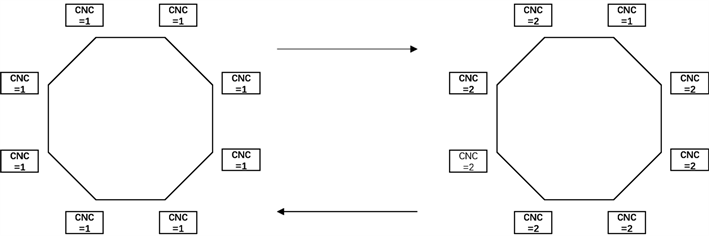注：CNC = 1表示在进行第一道工序的加工，CNC = 2表示在进行第二道工序的加工。

Figure 2. Cycle diagram (1)

2) 当y大于1，即第一道工序加工时间明显大于第二道工序加工时间，则选取所有CNC均在进行第一道工序的加工为循环节的初始状态，此时从第二道工序到第一道工序切换的优先级高于从第一道工序到第二道工序切换。经过如图3所示的调度过程，则最终一定会出现所有的CNC均进行第一道工序加工的状态，即出现了循环现象。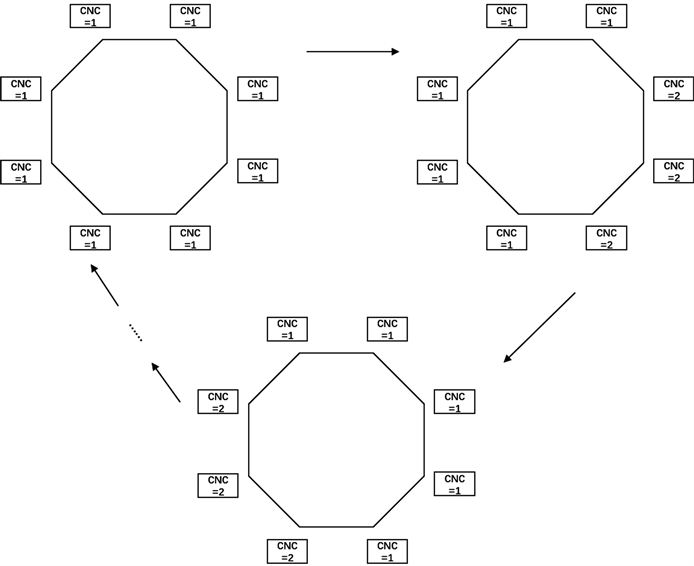Figure 3. Cycle diagram (2)

3) 当y小于1，即第一道工序加工时间明显小于第二道工序加工时间，则选取所有CNC均在进行第二道工序的加工为循环节的初始状态，RGV的调度情况与(2)同理，如图4所示，则一定会出现所有的CNC均进行第二道工序加工的状态，即出现了循环现象。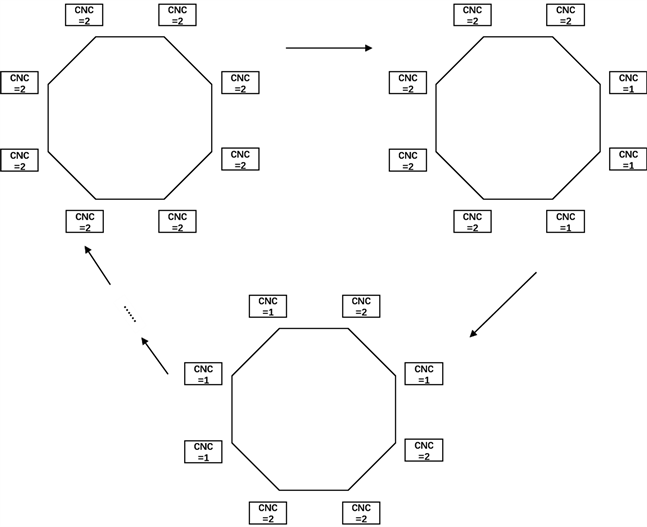Figure 4. Cycle diagram (3)

1) 当y近似于1，即两道工序所需时间大小相当时：

${T}_{\mathrm{min}}=\left\{\begin{array}{l}{t}_{2}+4\left({p}_{1}+{p}_{2}\right)+8q+\sum _{j=1}^{8}\sum _{i=1}^{8}{a}_{ij}{b}_{ij}+{p}_{1},\text{\hspace{0.17em}}\text{\hspace{0.17em}}循环起点为奇数号\text{CNC}\\ {t}_{2}+4\left({p}_{1}+{p}_{2}\right)+8q+\sum _{j=1}^{8}\sum _{i=1}^{8}{a}_{ij}{b}_{ij}+{p}_{2},\text{\hspace{0.17em}}\text{\hspace{0.17em}}循环起点为偶数号\text{CNC}\end{array}$ (1.7)

$\left\{\begin{array}{l}\sum _{i=1}^{8}{a}_{ij}=1,\text{\hspace{0.17em}}\text{\hspace{0.17em}}j=1,2,3,\cdots ,8\\ \sum _{j=1}^{8}{a}_{ij}=1,\text{\hspace{0.17em}}\text{\hspace{0.17em}}i=1,2,3,\cdots ,8\\ {a}_{ii}=0,\text{\hspace{0.17em}}\text{\hspace{0.17em}}i=1,2,3,\cdots ,8\\ \sum _{i,j\in s}{a}_{ij}\le |s|-1,\text{\hspace{0.17em}}\text{\hspace{0.17em}}2\le |s|\le 8-1,\text{\hspace{0.17em}}\text{\hspace{0.17em}}s\subset \left\{1,2,\cdots ,8\right\}\end{array}$ (1.8)

2) 当y小于1第一道工序所需时间明显小于第二道时：

$C\to A\to F\to H\to D\to C\to A\to H\to D\to B\to G\to E\to C\to A\to B\to G\to E$

$2×\left(AE+EG+GB\right)+BD+2×\left(AB+HF+FC\right)+3×AC+AB+CD$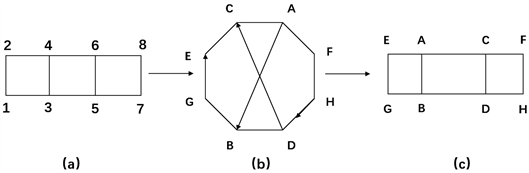Figure 5. Abstract route (1)

${T}_{\mathrm{min}}=4\left({p}_{1}+{p}_{2}\right)+8q+d$ (1.9)

$\left\{\begin{array}{l}|\sum _{i=1}^{8}{a}_{ij}-2|=0.5,\text{\hspace{0.17em}}\text{\hspace{0.17em}}j=1,2,3,\cdots ,8\\ \sum _{j=1}^{8}\sum _{i=1}^{8}{a}_{ij}=20\\ 4q+2\left({p}_{1}+{p}_{2}\right)+3{d}_{AB}\le {t}_{1}\\ 4q+2\left({p}_{1}+{p}_{2}\right)+3{d}_{CD}\le {t}_{1}\end{array}$ (1.10)

3) 当y大于1，即第一道工序所需时间明显大于第二道时：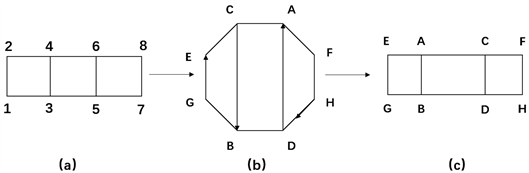Figure 6. Abstract route (2)

${T}_{\mathrm{min}}=4\left({p}_{1}+{p}_{2}\right)+6q+d$ (1.11)

$\left\{\begin{array}{l}|\sum _{i=1}^{8}{a}_{ij}-2|=0.5,\text{\hspace{0.17em}}\text{\hspace{0.17em}}j=1,2,3,\cdots ,8\\ \sum _{j=1}^{8}\sum _{i=1}^{8}{a}_{ij}=20\\ 2\left({p}_{1}+{p}_{2}\right)+{d}_{AB}\le {t}_{1}\\ 2\left({p}_{1}+{p}_{2}\right)+{d}_{CD}\le {t}_{1}\end{array}$ (1.12)

5.1.3. 基于时间分段的故障降维最优调度模型

CNC在加工过程中可能发生故障(据统计：故障的发生概率约为1%)的情况，每次故障排除(人工处理，未完成的物料报废)时间介于10~20分钟之间，故障排除后即刻加入作业序列。

${T}_{\mathrm{min}}=\mathrm{max}\left(560,\sum _{j=2}^{n}\sum _{i=1}^{n}{a}_{ij}{b}_{ij}+4{p}_{1}+3{p}_{2}+8q\right)+{p}_{2}+\sum _{i=1}^{8}{a}_{i1}{b}_{i1}$ (1.13)

$\left\{\begin{array}{l}\sum _{i=1}^{n}{a}_{ij}=1,\text{\hspace{0.17em}}\text{\hspace{0.17em}}j=1,2,3,\cdots ,n\\ \sum _{j=1}^{n}{a}_{ij}=1,\text{\hspace{0.17em}}\text{\hspace{0.17em}}i=1,2,3,\cdots ,n\\ {a}_{ii}=0,\text{\hspace{0.17em}}\text{\hspace{0.17em}}i=1,2,3,\cdots ,n\\ \sum _{i,j\in s}{a}_{ij}\le |s|-1,\text{\hspace{0.17em}}\text{\hspace{0.17em}}2\le |s|\le n-1,\text{\hspace{0.17em}}\text{\hspace{0.17em}}s\subset \left\{1,2,\cdots ,n\right\}\end{array}$ (1.14)

${T}_{\mathrm{min}}=\left\{\begin{array}{l}{t}_{2}+4\left({p}_{1}+{p}_{2}\right)+nq+\sum _{j=1}^{n}\sum _{i=1}^{n}{a}_{ij}{b}_{ij}+{p}_{1},\text{\hspace{0.17em}}\text{\hspace{0.17em}}循环起点为奇数号\text{CNC}\\ {t}_{2}+4\left({p}_{1}+{p}_{2}\right)+nq+\sum _{j=1}^{n}\sum _{i=1}^{n}{a}_{ij}{b}_{ij}+{p}_{2},\text{\hspace{0.17em}}\text{\hspace{0.17em}}循环起点为偶数号\text{CNC}\end{array}$ (1.15)

$\left\{\begin{array}{l}\sum _{i=1}^{n}{a}_{ij}=1,\text{\hspace{0.17em}}\text{\hspace{0.17em}}j=1,2,3,\cdots ,n\\ \sum _{j=1}^{n}{a}_{ij}=1,\text{\hspace{0.17em}}\text{\hspace{0.17em}}i=1,2,3,\cdots ,n\\ {a}_{ii}=0,\text{\hspace{0.17em}}\text{\hspace{0.17em}}i=1,2,3,\cdots ,n\\ \sum _{i,j\in s}{a}_{ij}\le |s|-1,\text{\hspace{0.17em}}\text{\hspace{0.17em}}2\le |s|\le n-1,\text{\hspace{0.17em}}\text{\hspace{0.17em}}s\subset \left\{1,2,\cdots ,n\right\}\end{array}$ (1.16)

5.2. 任务二模型的求解

5.2.1. 基于矩阵循环的最优调度模型求解

Step 1：初始化：将RGV的调度路线量化成 $8×8$ 的邻接矩阵A，将RGV在8个CNC之间移动的时间量化成位置矩阵B。

Step 2：求解目标函数：即最优路径的求解，根据RGV调度模型(1.4)数学规划的具体形式，最优路径的求解从根本上来看属于TSP问题，可以使用Lingo软件解决该类问题，从而得出一个循环周期内邻接矩阵A的具体表达形式。

Step 3：判断：利用Step 2中所得的邻接矩阵A，用Matlab软件模拟一个班次(即8小时)内RGV的循环调度过程，若当前上下料开始时间大于28,800秒，则退出循环。

Step 4：输出结果：输出一个班次内RGV的调度策略，从而得出该时段内生产工件的总数n。

Step 5：计算系统的作业效率：理想情况下，一个最短循环周期内完成8个工件的加工是最高效的，从而一个班次内最多可生产的工件数为：

${n}_{\mathrm{max}}=\frac{28800}{{T}_{\mathrm{min}}}×8$ (1.17)

$\eta =\frac{n}{{n}_{\mathrm{max}}}%$ (1.18)

$A=\left(\begin{array}{cccccccc}0& 0& 0& 0& 0& 0& 1& 0\\ 1& 0& 0& 0& 0& 0& 0& 0\\ 0& 0& 0& 1& 0& 0& 0& 0\\ 0& 1& 0& 0& 0& 0& 0& 0\\ 0& 0& 0& 0& 0& 1& 0& 0\\ 0& 0& 1& 0& 0& 0& 0& 0\\ 0& 0& 0& 0& 0& 0& 0& 1\\ 0& 0& 0& 0& 1& 0& 0& 0\end{array}\right)$ (1.19)注：t表示当前循环最后一个物料下料开始时间。

Figure 7. Algorithm flowchart of Model 1Table 2. Scheduling strategy of RGV and system operating efficiency

5.2.2. 基于时间决定因子的最优调度模型求解

Step 1：穷举路线：因为Lingo的局限性，采用穷举算法得到所有的路线排序。

Step 2：求解目标函数：根据约束条件，用穷举产生的所有排序求解目标函数，得到最优解，即是最优排序。

Step 3：判断：模拟一个班次(即8小时)内RGV的循环调度过程，若当前上下料开始时间大于28,800秒，则退出循环。

Step 4：输出结果：输出一个班次内RGV的调度策略，从而得出该时段内生产工件的总数n。

Step 5：计算系统的作业效率：理想情况下，一个最短循环周期内完成8个工件的加工是最高效的，从而一个班次内最多可生产的工件数和作业效率分别为：

${n}_{\mathrm{max}}=\frac{28800}{{T}_{\mathrm{min}}}×8$ (1.20)

$\eta =\frac{n}{{n}_{\mathrm{max}}}%$ (1.21)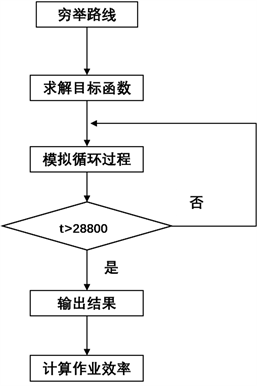Figure 8. Algorithm flowchart of Model 2Table 3. Scheduling strategy of RGV and system operating efficiency in two processes

5.2.3. 基于时间分段的故障降维最优调度模型求解

Step 1：初始化：将RGV的调度路线量化成 $8×8$ 的邻接矩阵A，将RGV在8个CNC之间移动的时间量化成位置矩阵B。

Step 2：班次时间分段：以1%为故障发生概率随机生成故障点，使故障点均匀的分布在n个加工成品之间，由故障点将一个班次时间分成若干片段。

Step 3：求解目标函数：对于无故障时间段，最优循环路径不变，对于故障段，对降维后的系统按照相应的最优调度模型求解，重新求解最优路径。

Step 4：判断：模拟一个班次(即8小时)内RGV的循环调度过程，若当前上下料开始时间大于28,800秒，则退出循环。

Step 5：输出结果：输出一个班次内RGV的调度策略，从而得出该时段内生产工件的总数n。

Step 6：计算系统的作业效率：理想情况下，一个最短循环周期内完成8个工件的加工是最高效的，从而一个班次内最多可生产的工件数和作业效率分别为：

${n}_{\mathrm{max}}=\frac{28800}{{T}_{\mathrm{min}}}×8$ (1.20)

$\eta =\frac{n}{{n}_{\mathrm{max}}}%$ (1.21)Figure 9. Algorithm flowchart of Model 3Table 4. Scheduling strategy of RGV and system operating efficiency in one process in case of failureTable 5. Scheduling strategy of RGV and system operating efficiency in two processes in case of failure

6. 总结

Dynamic Scheduling Strategy of Smart RGV Based on Matrix Cycle[J]. 应用数学进展, 2019, 08(03): 481-495. https://doi.org/10.12677/AAM.2019.83054

1. 1. Bhargava, A. 算法图解[M]. 袁国忠, 译. 北京: 人民邮电出版社, 2017.

2. 2. 司守奎, 孙玺菁. LINGO软件及应用[M]. 北京: 国防工业出版社, 2017.

3. 3. 张平. Matlab基础与应用[M]. 北京: 北京航空航天大学出版社, 2007.

4. 4. 史峰, 王辉. Matlab智能算法30个案例分析[M]. 北京: 北京航空航天大学出版社, 2011.

5. 5. 王燕军, 梁治安. 最优化基础理论与方法[M]. 上海: 复旦大学出版社, 2011.

6. 6. 张贤达. 矩阵分析与应用[M]. 第2版. 北京: 清华大学出版社, 2013.

NOTES

*第一作者。

#通讯作者。